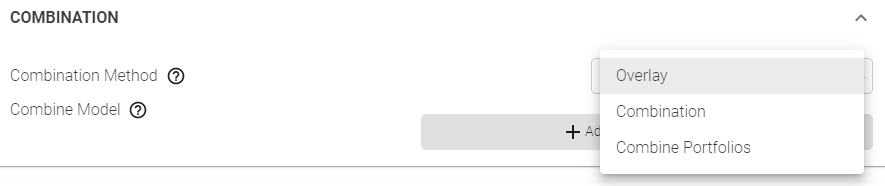# What's the difference between Overlay, Combination and Combine Portfolios?

When editing portfolio settings, in the Combination section, what is the difference between the three options: Overlay, Combination and Combine Portfolios?#### Overlay

• Overlay works on the concept of taking a core model and overlaying one or more models on top of those signals using an intersection
• It works on a “delta” basis. Where every pick in the models used to overlay the core model will be scored from -1 to 1 (with 1 being the best score).  You will alter the core model weights by a percentage of the delta based on that score.  So a score of 0.50 will modify the core model score by 12.5% if the delta is 25%.
• Here is a sample interaction:
• Core model has 9 stocks
• Overlay Model A with a 25% delta
• Overlay Model B with a 33% delta• You can see in this interaction that having a positive score from both models improves your score in Overlay A + B (i.e. AAPL goes from 0.100 to 0.146). Having one positive and one very negative score can lower your overall score though (i.e. GOOGL goes from 0.150 to 0.113).
• You can also see the AMZN is not in the core model (score = NaN, not a number) and even though it is in both Model A and Model B it remains out of the combined Overlay A + B universe.

#### Combination

• Combination works on the concept of taking a core model and combining one or more models with those signals using a union
• You set a weight for each model in your groupings of models (including the core model). The scores from each of the core models are converted from -1 to 1 and then multiplied by the weight.  The scores for each stock on each day are then added together.
• Here is a sample interaction:
• Core model has 9 stocks and a weight of 30%
• Model A has 10 stocks and a weight of 40%
• Model B has 8 stocks and a weight of 30%• It is harder to see in the table above – but the final score is just a reflection of all the adjusted scores, Core Adj. Score + Model A Adj. Score + Model B Adj. Score.
• Anywhere that a company is not in a universe it gets an adjusted score of 0.

#### Combine Portfolios

• Combine portfolios combines the post-optimized and constructed portfolios from 1 or more other portfolios. This means that it is using the resultant portfolio weights as input signals – and not the machine learning signals from the models underlying the portfolios.
• The math behind combining portfolios is identical to the Combination method – except portfolio weights are used and there is no need to scale the inputs -1 to 1. It is important to note that if two portfolios contain the same holding (i.e. AAPL at -10% in Portfolio A and +15% in Portfolio B, net weight will be +2.5% if both are 50% weight) that they will still be summed together and therefore the total allocation in the combined portfolio may be less than you expect.
• For example, if you had 50% weight a 100% long SP500 portfolio and 50% weight a 150% long and 50% short health care portfolio, the resultant portfolio might not be 125% long by 25% short. The net exposure will remain 100% but it might end up 120% long by 20% short due to the cancellation of some weights. -
• If you do not select simple portfolio combination, then the resultant portfolios will be added together into one portfolio and respect the portfolio settings you select (i.e. optimizer, long %, short %, etc). If you do select simple portfolio combination then all that happens is the two (or more) portfolios get added together and the portfolio settings for the main portfolio will not be respected.
• Finally, it is important to note that you can combine portfolios from any model (currently). The combine portfolios function uses only the portfolios specified in the dialogue.  It will completely ignore the model/portfolio that it is attached to.  So you could have a “combine portfolio” that started in a US energy model but uses a Canadian Financial portfolio + a US Health Care portfolio and the results will only use Canadian Financials + US Health Care.